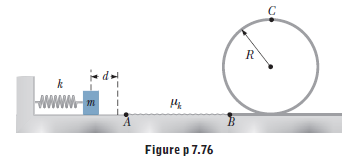# Problem: A massless spring of constant k = 78.4 N/m is fixed on the left side of a level track. A block of mass m = 0.50 kg is pressed against the spring and compresses it a distance d, as in Figure P7.76. The block (initially at rest) is then released and travels toward a circular loop-the-loop of radius R = 1.5 m. The entire track and the loop-the-loop are frictionless, except for the section of track between points A and B. Given that the coefficient of kinetic friction between the block and the track along AB is μk = 0.30, and that the length of AB is 2.5 m, determine the minimum compression d of the spring that enables the block to just make it through the loop-the-loop at point C. Hint: The force exerted by the track on the block will be zero if the block barely makes it through the loop-the-loop.

⚠️Our tutors found the solution shown to be helpful for the problem you're searching for. We don't have the exact solution yet.

###### Problem Details

A massless spring of constant k = 78.4 N/m is fixed on the left side of a level track. A block of mass m = 0.50 kg is pressed against the spring and compresses it a distance d, as in Figure P7.76. The block (initially at rest) is then released and travels toward a circular loop-the-loop of radius R = 1.5 m. The entire track and the loop-the-loop are frictionless, except for the section of track between points A and B. Given that the coefficient of kinetic friction between the block and the track along AB is μk = 0.30, and that the length of AB is 2.5 m, determine the minimum compression d of the spring that enables the block to just make it through the loop-the-loop at point C. Hint: The force exerted by the track on the block will be zero if the block barely makes it through the loop-the-loop.